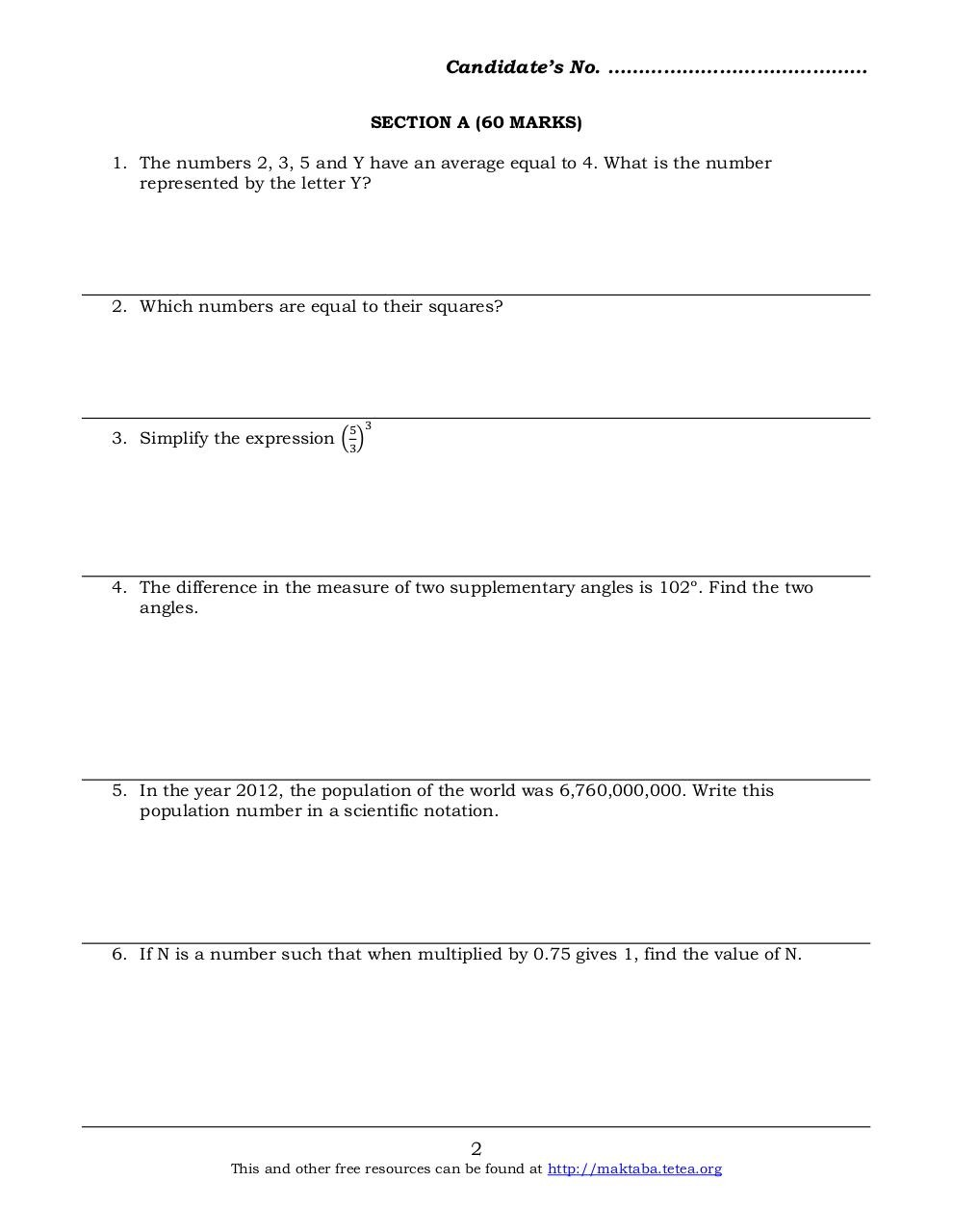# Basic Math 2013 F2.pdfPage 1 2 3 4 5 6 7 8

#### Text preview

Candidate’s No. ……………………………………
SECTION A (60 MARKS)
1. The numbers 2, 3, 5 and Y have an average equal to 4. What is the number
represented by the letter Y?

2. Which numbers are equal to their squares?

5 3

3. Simplify the expression (3)

4. The difference in the measure of two supplementary angles is 102º. Find the two
angles.

5. In the year 2012, the population of the world was 6,760,000,000. Write this
population number in a scientific notation.

6. If N is a number such that when multiplied by 0.75 gives 1, find the value of N.

2

This and other free resources can be found at http://maktaba.tetea.org